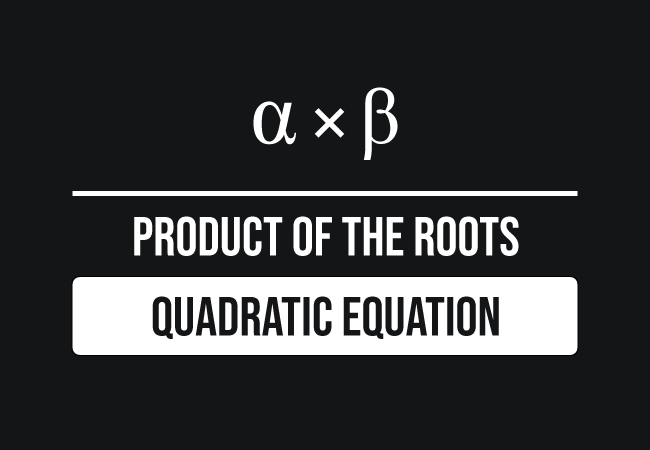# Product of Roots of a Quadratic equation

## Formula

$\alpha .\beta \,=\, \dfrac{c}{a}$

### Introduction

The product by multiplying the two roots of a quadratic equation is called the product of the roots of a quadratic equation.In mathematics, a quadratic equation is written as $ax^2+bx+c$ $\,=\,$ $0$ in general form. So, it has two solutions and let’s denote the two roots by two Greek alphabets $\alpha$ and $\beta$.

$\alpha$ $\,=\,$ $\dfrac{-b+\sqrt{b^2\,-\,4ac}}{2a}$

$\beta$ $\,=\,$ $\dfrac{-b\,-\sqrt{b^2\,-\,4ac}}{2a}$

Now, let us multiply them to find the product of the zeros of a quadratic equation.

$\implies$ $\alpha$ $\times$ $\beta$ $\,=\,$ $\Bigg(\dfrac{-b+\sqrt{b^2\,-\,4ac}}{2a}\Bigg)$ $\times$ $\Bigg(\dfrac{-b-\sqrt{b^2\,-\,4ac}}{2a}\Bigg)$

There are two fractions on the right hand side of the equation and they are involved in the multiplication. So, let us focus on finding the product by multiplying them.

$\,\,=\,\,$ $\dfrac{\big(-b+\sqrt{b^2\,-\,4ac}\big) \times \big(-b-\sqrt{b^2\,-\,4ac}\big)}{2a \times 2a}$

Look at the denominator firstly. A factor $2a$ is multiplied by itself and let’s write their product.

$\,\,=\,\,$ $\dfrac{\big((-b)+\sqrt{b^2\,-\,4ac}\big) \times \big((-b)-\sqrt{b^2\,-\,4ac}\big)}{4a^2}$

Now, look at the factors at the numerator. Each factor is a binomial and their terms of both factors are same but they have opposite signs. So, the product of them represents the expansion of the difference of squares. Now, let’s simplify the product by the difference of squares rule.

$\,\,=\,\,$ $\dfrac{\big(-b\big)^2-\big(\sqrt{b^2\,-\,4ac}\big)^2}{4a^2}$

It is time to simplify the expression in the numerator to find its value.

$\,\,=\,\,$ $\dfrac{b^2-\big(b^2\,-\,4ac\big)}{4a^2}$

$\,\,=\,\,$ $\dfrac{b^2-b^2+4ac}{4a^2}$

$\,\,=\,\,$ $\dfrac{\cancel{b^2}-\cancel{b^2}+4ac}{4a^2}$

$\,\,=\,\,$ $\dfrac{4ac}{4a^2}$

$\,\,=\,\,$ $\dfrac{4a \times c}{4a \times a}$

$\,\,=\,\,$ $\dfrac{\cancel{4a} \times c}{\cancel{4a} \times a}$

$\,\,\,\therefore\,\,\,\,\,\,$ $\alpha .\beta \,=\, \dfrac{c}{a}$

##### Example

$3x^2-4x+7 \,=\, 0$

Let’s learn how to find the product of the roots of a quadratic equation. Firstly, compare the quadratic equation with the standard form of a quadratic equation. Now, the values of $a = 3$, $b = -4$ and $c = 7$.

$\,\,\,\therefore\,\,\,\,\,\,$ $\dfrac{c}{a} \,=\, \dfrac{7}{3}$

###### Recommended
Latest Math Topics
Jun 26, 2023
Jun 23, 2023

Latest Math Problems
Jul 01, 2023
Jun 25, 2023
###### Math Questions

The math problems with solutions to learn how to solve a problem.

Learn solutions

Practice now

###### Math Videos

The math videos tutorials with visual graphics to learn every concept.

Watch now

###### Subscribe us

Get the latest math updates from the Math Doubts by subscribing us.